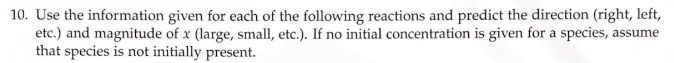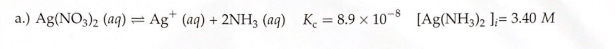# 10. Use the information given for each of the following reactions and predict the direction (right, left, etc.) and magnitude of x (large, small, etc). If no initial concentration is given for a species, assume that species is not initially present. a.) Ag(NO3)2 (aq) ⇌ Ag^+ (aq) + 2NH3 (aq) Kc = 8.9 X 10^-8 [Ag(NH302]i= 3.40 M